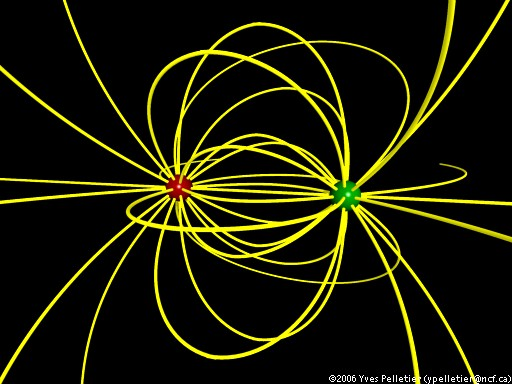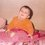# DielectricsI am really confused about the behaviour of electric field through dielectrics.

I have a question:

Let's suppose that I have two point charges, $q_1$ and $q_2$, separated by free space. Now the force of attraction between them is clearly obtained by coulombs law, ie:

$\large F = \dfrac{q_1 q_2}{4\pi \epsilon_0 r^2}$

Now suppose that instead of free space, the whole system is in a dielectric, then again we clearly conclude to:

$\large F = \dfrac{q_1 q_2}{4\pi \epsilon_0 k r^2},$

where $k$ is the dielectric constant of the dielectric in which the system is contained.

Now suppose that the separation between the two point charges is partially free space and partially the dielectric s.t. none of the charges is contained in the dielectric, then:

• As I have seen in some books, they treat the distance d of dielectric as $d \sqrt {k}$ and then the equation they use is:

$\large F = \dfrac{q_1 q_2}{4\pi \epsilon_0(r - d + d \sqrt k)^2}$

I am a bit doubtful about this relation. As this would imply that a metal sheet would completely prevent the two particles from interacting with each other which is not the case.

• The second thing which i feel is that the reduction in electric field in a dielectric is basically due to an opposing field developed in the dielectric. And hence the answer to electric field at a point due to a charge would depend only on the medium in which it (the point) is present.

I feel that there is a grave error in my concepts.

Thanks and regards: PrabhpreetNote by Prabhpreet Sodhi
5 years, 2 months ago

This discussion board is a place to discuss our Daily Challenges and the math and science related to those challenges. Explanations are more than just a solution — they should explain the steps and thinking strategies that you used to obtain the solution. Comments should further the discussion of math and science.

When posting on Brilliant:

• Use the emojis to react to an explanation, whether you're congratulating a job well done , or just really confused .
• Ask specific questions about the challenge or the steps in somebody's explanation. Well-posed questions can add a lot to the discussion, but posting "I don't understand!" doesn't help anyone.
• Try to contribute something new to the discussion, whether it is an extension, generalization or other idea related to the challenge.

MarkdownAppears as
*italics* or _italics_ italics
**bold** or __bold__ bold
- bulleted- list
• bulleted
• list
1. numbered2. list
1. numbered
2. list
Note: you must add a full line of space before and after lists for them to show up correctly
paragraph 1paragraph 2

paragraph 1

paragraph 2

[example link](https://brilliant.org)example link
> This is a quote
This is a quote
    # I indented these lines
# 4 spaces, and now they show
# up as a code block.

print "hello world"
# I indented these lines
# 4 spaces, and now they show
# up as a code block.

print "hello world"
MathAppears as
Remember to wrap math in $$ ... $$ or $ ... $ to ensure proper formatting.
2 \times 3 $2 \times 3$
2^{34} $2^{34}$
a_{i-1} $a_{i-1}$
\frac{2}{3} $\frac{2}{3}$
\sqrt{2} $\sqrt{2}$
\sum_{i=1}^3 $\sum_{i=1}^3$
\sin \theta $\sin \theta$
\boxed{123} $\boxed{123}$

Sort by:

Yes the metal sheet will prevent the two charges from interacting because as soon as you place the charges in the sheet the charges will neutralise by free electrons present in the sheet because there cannot be an electric field in the sheet in electrostatic condition (because if there is field then electrons will move and it will no longer be electrostatic condition, it will be electrodynamic condition)

- 5 years, 2 months ago

Saurav, suppose i have a positive charge placed inside a metal shell. Now the field lines from the charge enter the shell and leave it from the outer surface. Since elofs are emerging it means that e field due to that charge at a point outside the metal shell is some finite value.

- 5 years, 2 months ago

No, as soon as you place the positive charge inside, there is E field in the metal and as metal contains free electrons therefore there is force on electrons toward the +ve charge and as the electrons move from some part of shell to your placed +ve charge, so $e^-$ deficiency occurs at that part of shell which is infact +ve charge ($e^-$ deficiency = +ve charge)

The process continues till you finally get zero net field inside the shell so that $e^-$ don't move further

- 5 years, 2 months ago